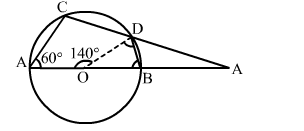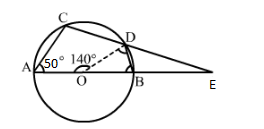# In the given figure, O is the centre of a circle.

Question:

In the given figure, O is the centre of a circle. If AOD = 140° and ∠CAB = 50°, calculate
(i) ∠EDB,
(ii) ∠EBD.Solution:O is the centre of the circle where AOD = 140° and CAB = 50°.
(i) BOD = 180° – AOD
= (180° – 140°) = 40°
We have the following:
OB = OD (Radii of a circle)
OBD = ODB

In ΔOBD, we have:
BOD + OBD + ODB = 180°
⇒ BOD + OBD + OBD = 180°      [∵ OBD = ODB]
⇒ 40° +2OBD = 180°
⇒ 2OBD = (180° – 40°) = 140°
⇒ OBD = 70°
Since ABCD is a cyclic quadrilateral, we have:
CAB + BDC = 180°
⇒ CAB + ODB ODC = 180°
⇒ 50° + 70° + ODC = 180°
⇒ ODC = (180° – 120°) = 60°
∴ ODC = 60°
EDB = (180° – (ODC + ODB)
= 180° – (60° + 70°)
= 180° – 130° = 50°
∴ EDB = 50°

(ii) EBD = 180° - ∠OBD
= 180° - 70°
= 110°